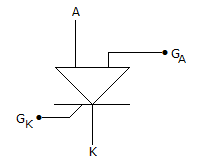# Electronics and Communication Engineering - Power Electronics

Exercise : Power Electronics - Section 4
31.
In a thyristors are i = kva. The value of a is
Explanation:
No answer description is available. Let's discuss.

32.
In an ordinary thyristor
turn on and turn off times are equal
turn on time is more than turn off time
turn off time is more than turn on time
turn on time may be equal, less than or more than turn off time
Explanation:
No answer description is available. Let's discuss.

33.
Thyristors connected in series need :
static equalizing circuit
dynamic equalizing circuit
both static and dynamic equalizing circuits
none of the above
Explanation:
No answer description is available. Let's discuss.

34.
The symbol in figure is forSUS
SBS
SCS
None of the above